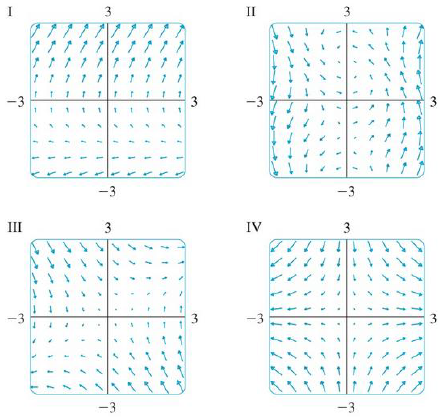Chapter 16.1, Problem 12E

Chapter
Section
Textbook Problem

Match the vector fields F with the plots labeled I-IV. Give reasons for your choices.12. F(x, y) = ⟨y, x − y⟩To determine

To match: The vector field F(x,y)=y,xy with the plots labeled as I-IV.

Explanation

Given data:

The vector field F(x,y)=y,xy.

Formula used:

The expression for the length of the two-dimensional vector F=x,y,

|F(x,y)|=x2+y2 (1)

Find the length of F(x,y) using equation (1).

|F(x,y)|=(y)2+(xy)2

Consider a certain interval of x as (2,2) and y as (2,2) to plot F(x,y).

The estimated values of |F(x,y)| and F(x,y) for different values of x and y are shown in Table 1.

Table 1

 Quadrant (x,y) |F(x,y)|=(y)2+(x−y)2 F(x,y)=〈y,x−y〉 I (0,0) 0 〈0,0〉 (1,0) 1 〈0,1〉 (2,0) 2 〈0,2〉 (0,1) 2 〈1,−1〉 (1,1) 1 〈1,0〉 (0,2) 22 <

Still sussing out bartleby?

Check out a sample textbook solution.

See a sample solution

The Solution to Your Study Problems

Bartleby provides explanations to thousands of textbook problems written by our experts, many with advanced degrees!

Get Started

Convert the expressions in Exercises 6584 to power form. 35x2

Finite Mathematics and Applied Calculus (MindTap Course List)

Other Equations Find all real solutions of the equation. 94. 8x1=3

Precalculus: Mathematics for Calculus (Standalone Book)